# 机器学习基石-数学篇：When Can Machines Learn?

## Coursera | Machine Learning Foundations - Mathematical Foundations | Hsuan-Tien Lin

Thistledown    June 30, 2020

## 1 The Learning Problem

What machine learning is and its connection to applications and other fields …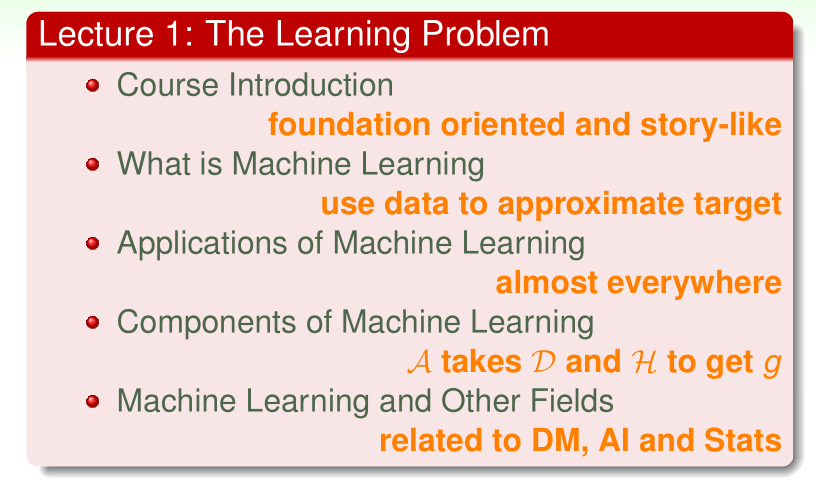#### 1.1 Course Introduction

• Machine Learning: a mixture of theoretical and practical tools

• theory oriented
• derive everything deeply for solid understanding
• less interesting to general audience
• techniques oriented
• flash over the sexiest techniques broadly for shiny converge
• too many techniques, hard to choose, hard to use properly
• **Our approach: **foundation oriented
• Foundation Oriented ML Course

• mixture of philosophical illustrations, key theory, core techniques, usage in practice and hopefully jokes

—- what every machine learning user should know

• story-like:

• When Can Machines Learn? (illustrative + technical)
• Why Can Machines Learn? (theoretical + illustrative)
• How Can Machines Learn? (technical + practical)
• How Can Machines Learn Better? (practical + theoretical)
• allows students to learn “future/untaught” techniques or study deeper theory easily

#### 1.2 What is Machine Learning

• From Learning to Machine Learning

• learning: acquiring skill with experience accumulated from observations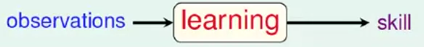• machine learning: acquiring skill with experience accumulated/computed from data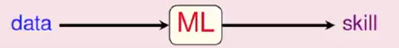• what is skill ?

• improve some performance measure (e.g. prediction accuracy)
• A more concrete definition:

• machine learning: improving some performance measure with experience computed from data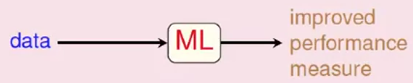• For example:• Yet another application: tree recognition

• “define” trees and hand-program: difficult
• learn from data (observations) and recognize: a 3-year-old can do so
• “ML-based tree recognition system” can be easier to build than hand-programmed system
• The machine learning route

• ML: an alternative route to build complicated systems
• Some use scenarios:
• When human cannot program the system manually:
• navigating on Mars
• When human cannot “define the solution” easily:
• speech/visual recognition
• When needing rapid decisions that humans cannot do
• When needing to be user-oriented in a massive scale
• consumer-targeted marketing
•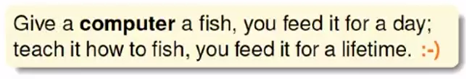• Key essence of ML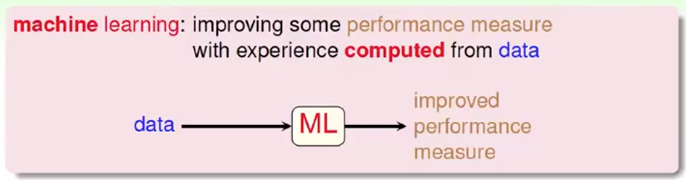• exists some "underlying pattern" to be learned
• so “performance measure” can be improved
• but no programmable (easy) definition
• so “ML” is needed
• somehow there is data about the pattern
• so ML has some “inputs” to learn
QUIZ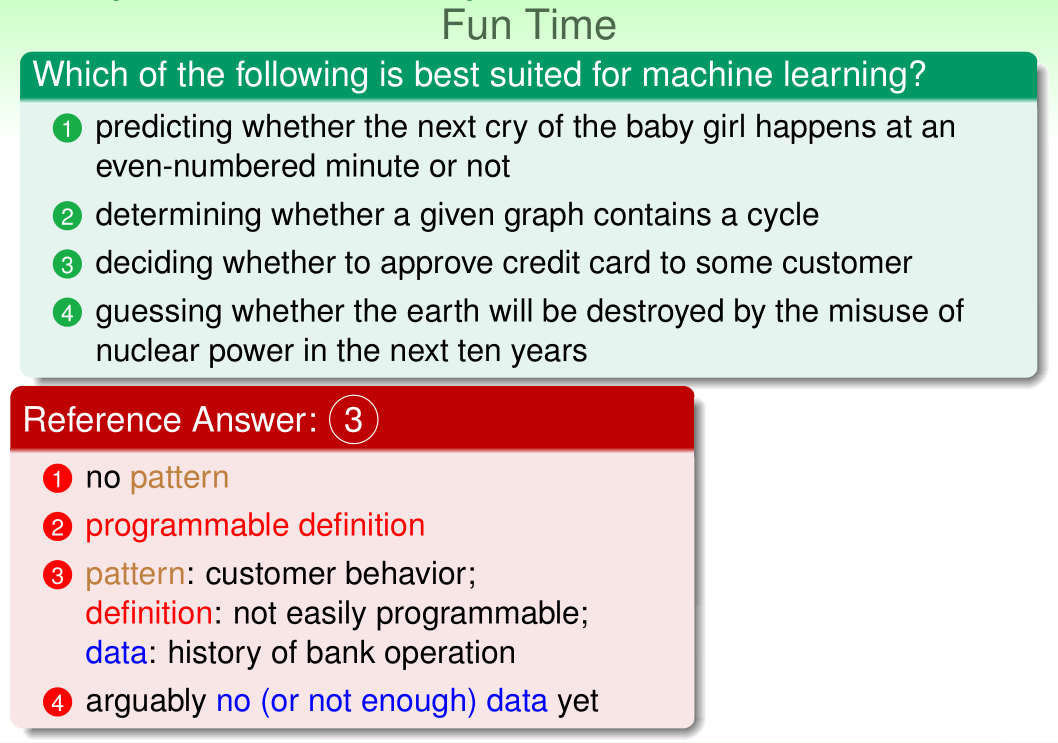#### 1.3 Applications of Machine Learning

• Daily needs: food, clothing, housing, transportation• Food (Sadilek et al., 2013)
• data: twitter data (words + location)
• skill: tell food poisoning likeliness of restaurant properly
• Clothing (Abu-Mostafa, 2012)
• data: sales figures + client surveys
• skill: give good fashion recommendations to clients
• Housing: (Tsanas and Xifara, 2012)
• data: characteristics of buildings and their energy load
• skill: predicted energy load of other buildings closely
• Transportation: (Stallkamp et al., 2012)
• data: some traffic sign images and meanings
• skill: recognize traffic signs accurately
• And more:

• Education

• data: students’ records on quizzes on a Math tutoring system
• skill: predict whether a student can give a correct answer to another quiz question
• A possible ML solution: answer correctly $\approx$ [recent strength of students $>$ difficulty of question]
• give ML 9 million records from 3,000 students
• ML determines (reverse-engineers) strength and difficulty automatically
• Entertainment: recommender system

• data: how many users have rated some movies

• skill: predict how a user would rate an unrated movie

• A hot problem:

• competition held by Netflix in 2006
• 100,480,507 ratings that 480,189 users gave to 17,770 movies
• 10% improvement = 1 million dollar prize
• similar competition (movies → songs) held by Yahoo! in KDDCup 2011
• 252,800,275 ratings that 1,000,990 users gave to 624,961 songs
• A possible ML solution: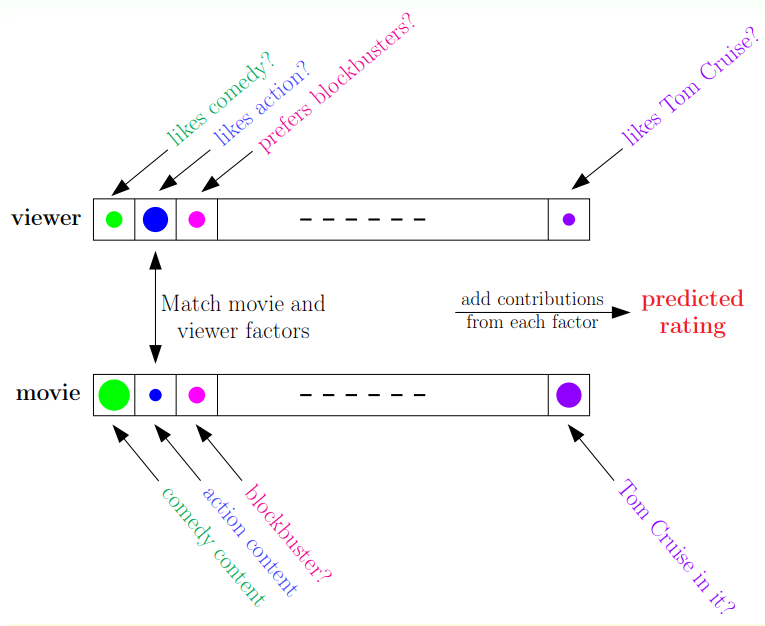• pattern: rating ← viewer/movie factors
• learning: known rating
• learned factors
• unknown rating prediction
QUIZ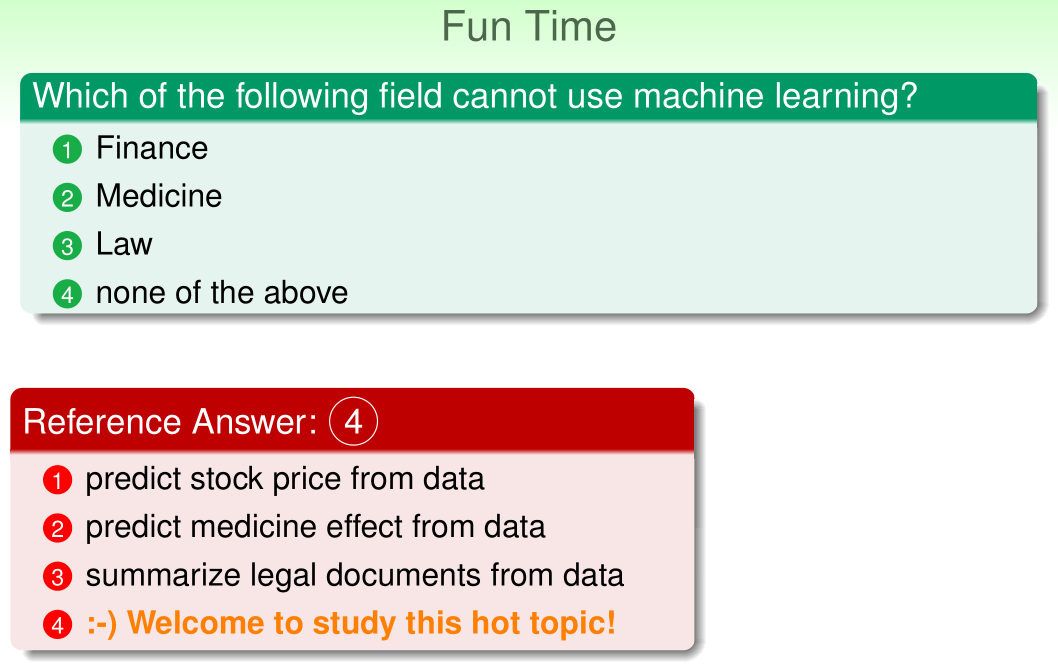#### 1.4 Components of Machine Learning

Metaphor using credit approval

• Application information: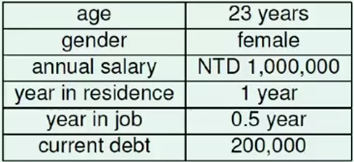• Unknown pattern to be learned: “approve credit card good for bank?”

• Formalize the learning problem:

• basic notations:• input: $\bf{x} \in \cal{X}$ (customer application)
• output: $y \in \cal{Y}$ (good/bad after approving credit card)
• unknown pattern to be learned $\Leftrightarrow$ target function: $f: \cal{X} \rightarrow \cal{Y}$ (ideal credit approval formula)
• data $\Leftrightarrow$ training examples: $\mathcal{D} = \left\lbrace (\mathbf{x}_1, y_1), \mathbf{x}_2, y_2), \dots, \mathbf{x}_N, y_N) \right\rbrace$ (historical records in bank)
• hypothesis $\Leftrightarrow$ skill with hopefully good performance: $g: \mathcal{X} \rightarrow \mathcal{Y}$ (“learned” formula to be used)
• learning flow to credit approval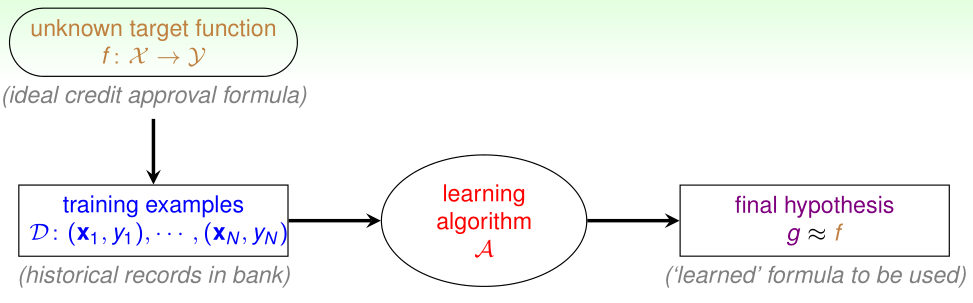• target $f$ unknown (i.e. no programmable definition)
• hypothesis $g$ hopefully $\approx f$, but possibly different from $f$ (perfection “impossible” when $f$ unknown)
• What does $g$ look like?
• The learning model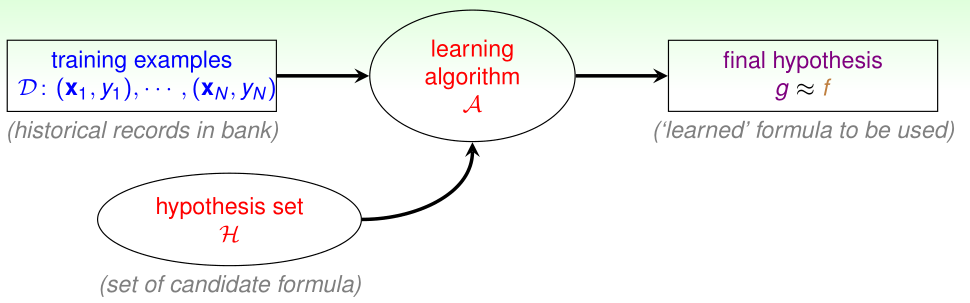• assume $g \in \mathcal{H} =\lbrace h_k \rbrace$, i.e. approving if
• $h_1$: annual salary > $800,000 •$h_2$: debt >$100,000 (really?)
• $h_3$: year in job $\le$ 2 (really?)
• hypothesis set $\cal H$:
• can contain good or bad hypothesis
• Practical definition of machine learning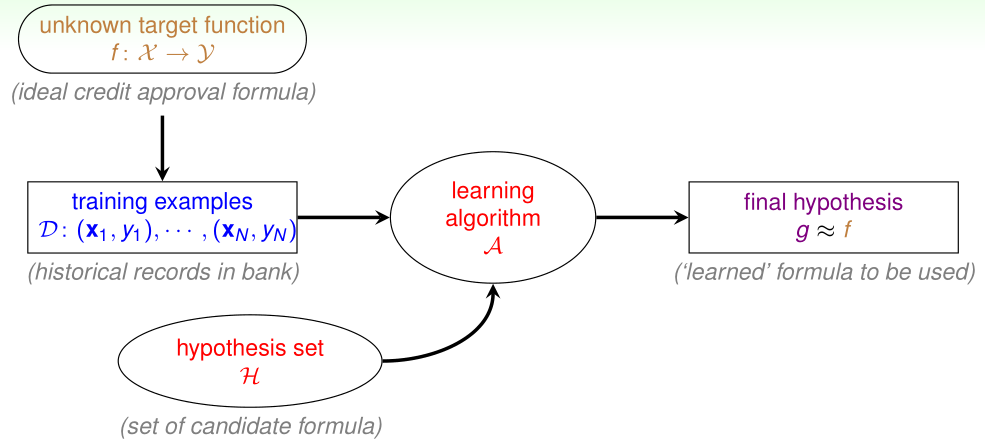• use data to compute hypothesis $g$ that approximates target $f$
QUIZ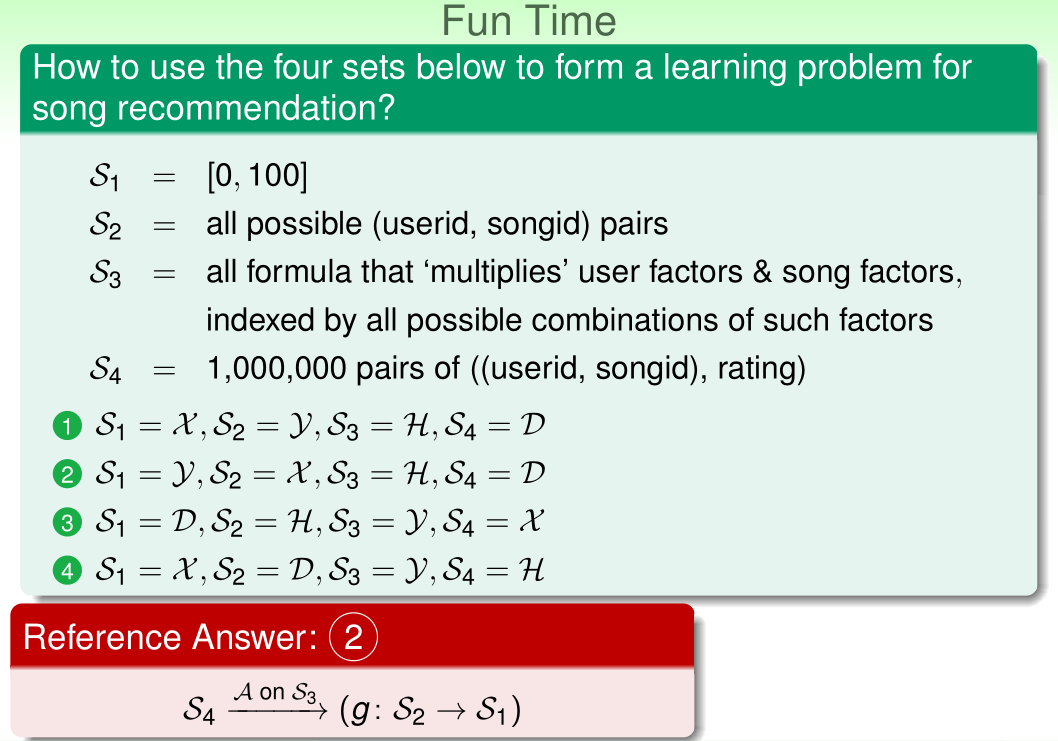#### 1.5 Machine Learning and Other Fields

• Machine Learning and Data Mining
• Definition
• Machine learning use data to compute hypothesis $g$ that approximates target $f$
• Data mining use (huge) data to find property that is interesting
• if “interesting property” same as “hypothesis that approximate target”
• ML = DM
• if “interesting property” related to “hypothesis that approximate target”
• DM can help ML, and vice versa (often, but not always)
• traditional DM also focuses on efficient computation in large database
• difficult to distinguish ML and DM in reality
• Machine Learning and Artificial Intelligence
• Definition
• Machine learning use data to compute hypothesis $g$ that approximates target $f$
• Artificial Intelligence compute something that shows intelligent behavior
• $g \approx f$ is something that shows intelligent behavior
• ML can realize AI, among other routes
• e.g. chess playing
• ML for AI: “learning from board data”
• ML is one possible route to realize AI
• Machine Learning and Statistics
• Definition
• Machine learning use data to compute hypothesis $g$ that approximates target $f$
• Statistics use data to make inference about an unknown process
• $g$ is an inference outcome; $f$ is something unknown
• Statistics can be used to achieve ML
• traditional statistics also focus on provable results with math assumptions, and care less about computation
• Statistics: many useful tools for ML
QUIZ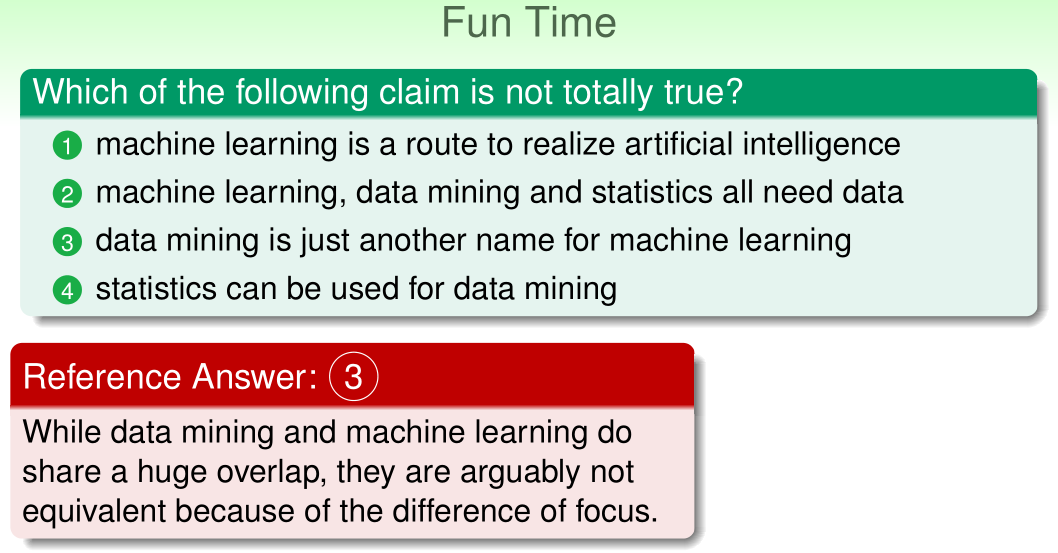## 2 Learning to Answer Yes/No

Your first learning algorithm (and the world’s first!) that “draws the line” between yes and no by adaptively searching for a good line based on data …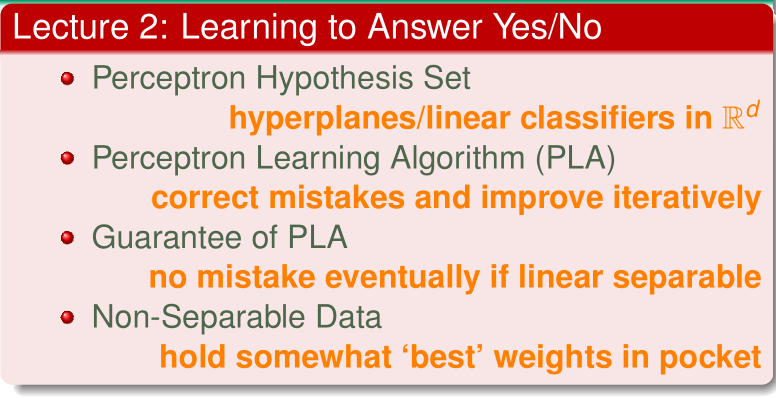#### 2.1 Perceptron Hypothesis Set

• A simple hypothesis set: the “Perceptron”

• for $\mathbf{x} = (x_1, x_2, \dots, x_d)$ “feature of customer”, compute a weighted “score” and: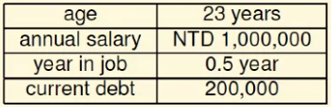• approve credit if $\sum_{i=1}^d w_ix_i > \text{threshold}$
• deny credit if $\sum_{i=1}^d w_ix_i < \text{threshold}$
• $\mathcal{Y}:\lbrace +1(\text{good}), -1(\text{bad}) \rbrace$, (0 ignored) - linear formula $h \in \cal{H}$ are:

• called perceptron hypothesis historically
• Vector form of perceptron hypothesis

• each “tall” $\mathbb{w}$ represents a hypothesis $h$ & is multiplied with “tall” $\mathbf{x}$ – will use tall version to simplify notation
• what do perceptron $h$ “look like”?
• Perceptron in $\Bbb{R}^2$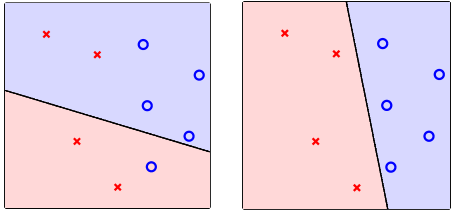• $h(\mathbf{x}) = \text{sign}(\mathbf{w}^T\mathbf{x}) = \text{sign}(w_0+w_1x_1+w_2x_2)$

• customer features $\mathbf{x}$: points on the plane (or points in $\Bbb{R}^d$)

• labels $y$: $\color{blue}{\circ (+1)}, \color{red}{\times (-1)}$

• hypothesis $h$: lines (or hypothesis in $\Bbb{R}^d$)

• positive on one side of a line, negative on the other side

QUIZ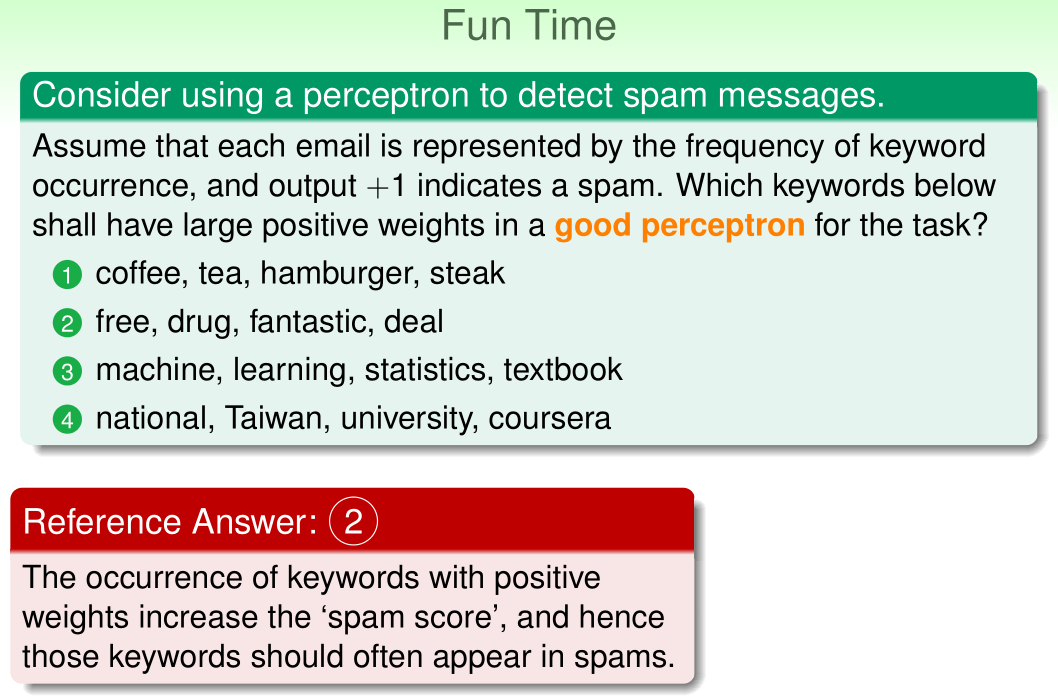#### 2.2 Perceptron Learning Algorithm (PLA)

• Select $g$ from $\cal{H}$

• $\cal H$ = all possible perceptrons, $g = ?$

• want: $g \approx f$ (hard when $f$ unknown)

• almost necessary: $g \approx f$ on $\cal D$, ideally $g(\mathbf{x}_n) = f(\mathbf{x}_n)=y_n$

• difficult: $\cal H$ is of infinite size

• idea: start from some $g_0$, and “correct” its mistakes on $\cal D$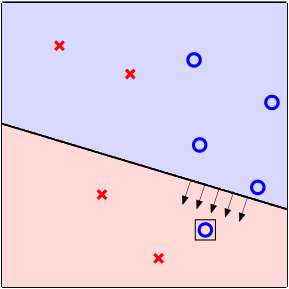• will present $g_0$ by its weight vector $\mathbf{w}_0$

• Perceptron Learning Algorithm

• start from some $\mathbf{w}_0$ (say, $\mathbf{0}$), and “correct” its mistakes on $\cal D$

• For $t = 0, 1, \dots$

• find a mistake of $\mathbf{w}t$, called $(x{n(t)}, y_{n(t)})$, and $\text{sign}\left( \mathbf{w}t^T\mathbf{x}{n(t)} \right) \ne y_{n(t)}$

• (try to) correct the mistake by $\mathbf{w}{t+1} \leftarrow \mathbf{w}_t + y{n(t)}x_{n(t)}$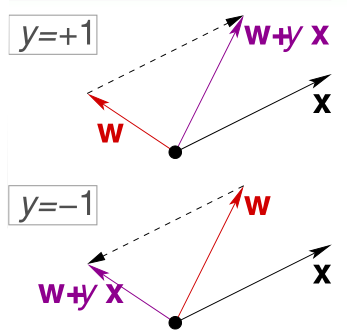• … until no more mistakes

• return last $\mathbf{w}$ called $\mathbf{w}_{PLA}$ as $g$

• Cyclic PLA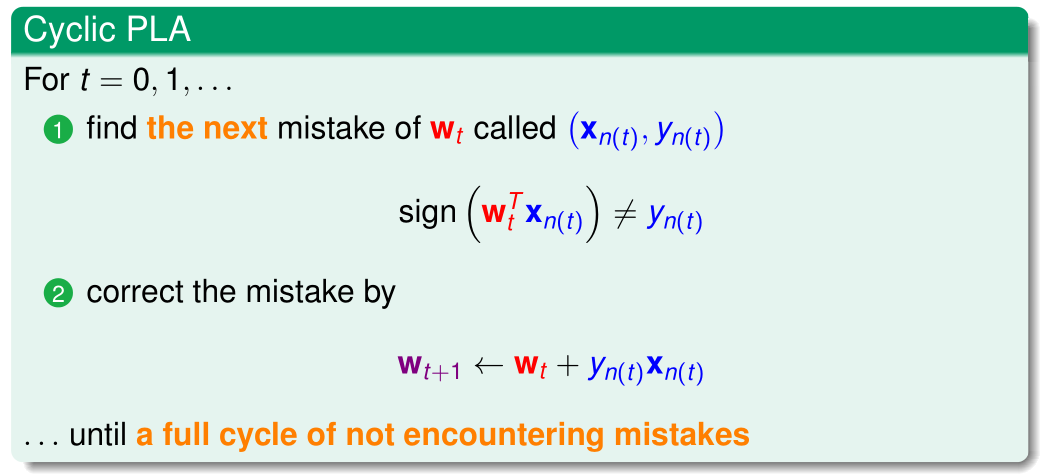• next can follow naïve cycle $(1, \dots, N)$ or precomputed random cycle
• Some remaining issues of PLA

• “correct” mistakes on $\cal D$ until no mistakes
• Algorithmic: halt (with no mistakes)?
• naïve cyclic: ??
• random cyclic: ??
• other variant: ??
• Learning: $g \approx f$ ?
• on $\cal D$, if halt, yes (no mistake)
• outside $\cal D$: ??
• if not halting: ??
• if (…), after enough corrections, any PLA variant halts
QUIZ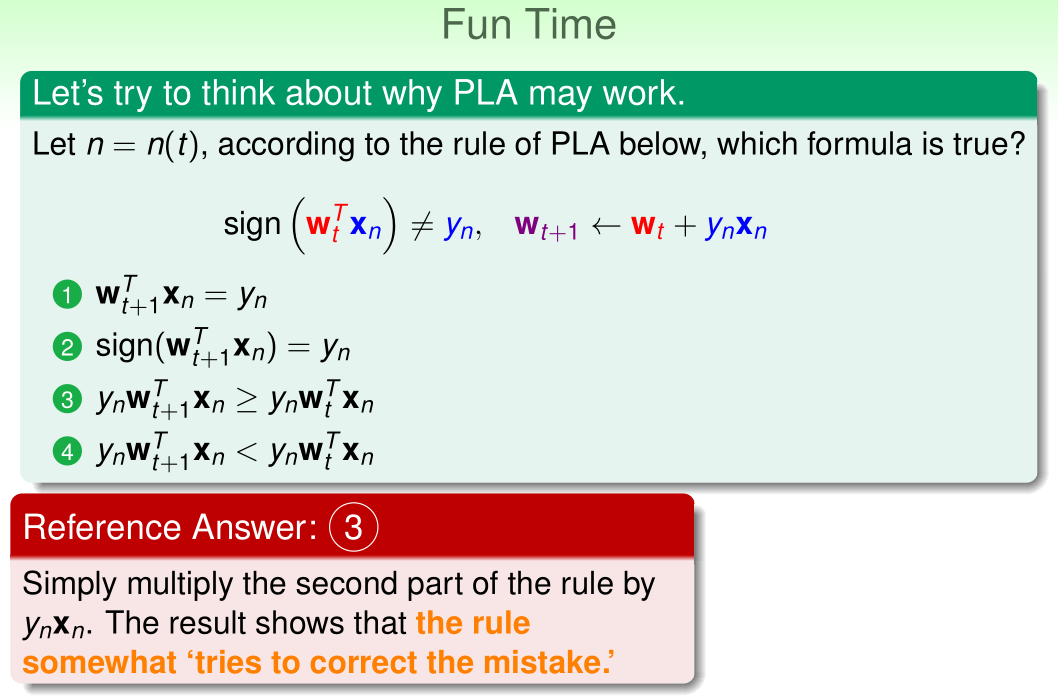#### 2.3 Guarantee of PLA

• Linear separability

• if PLA halts (i.e. no more mistakes)
• (necessary condition) $\cal {D}$ allows some $\bf w$ to make no mistake
• call such $\cal D$ linear separable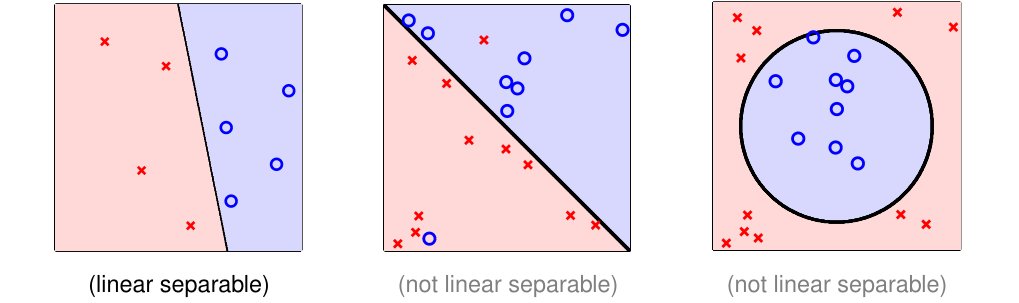• assume linear separable $\cal D$, dose PLA always halt?
• PLA fact: $\mathbf{w}_t$ gets more aligned with $\mathbf{w}_f$

• linear separable $\Leftrightarrow$ exists perfect $\mathbf{w}_f$ such that $y_n = \text{sign}(\mathbf{w}_f^T\mathbf{x}_n)$

• $\mathbf{w}_f$ perfect hence every $\mathbf{x}_n$ correctly away from line:

• by updating with any $\left(\mathbf{x}{n(t)}, y{n(t)}\right)$,

• PLA fact: $\mathbf{w}_t$ does not grow too fast

• $\mathbf{w}t$ change only when mistake $\Leftrightarrow$ $\text{sign}(\mathbf{w}_t^T\mathbf{x}{n(t)}) \ne y_{n(t)}$ $\Leftrightarrow$ $y_{n(t)}\mathbf{w}t^T\mathbf{x}{n(t)} \le 0$

• mistake limits $|\mathbf{w}_t|^2$ growth, even when updating with longest $\mathbf{x}_n$

• start from $\mathbf{w}_0=0$, after $T$ mistake corrections,

QUIZ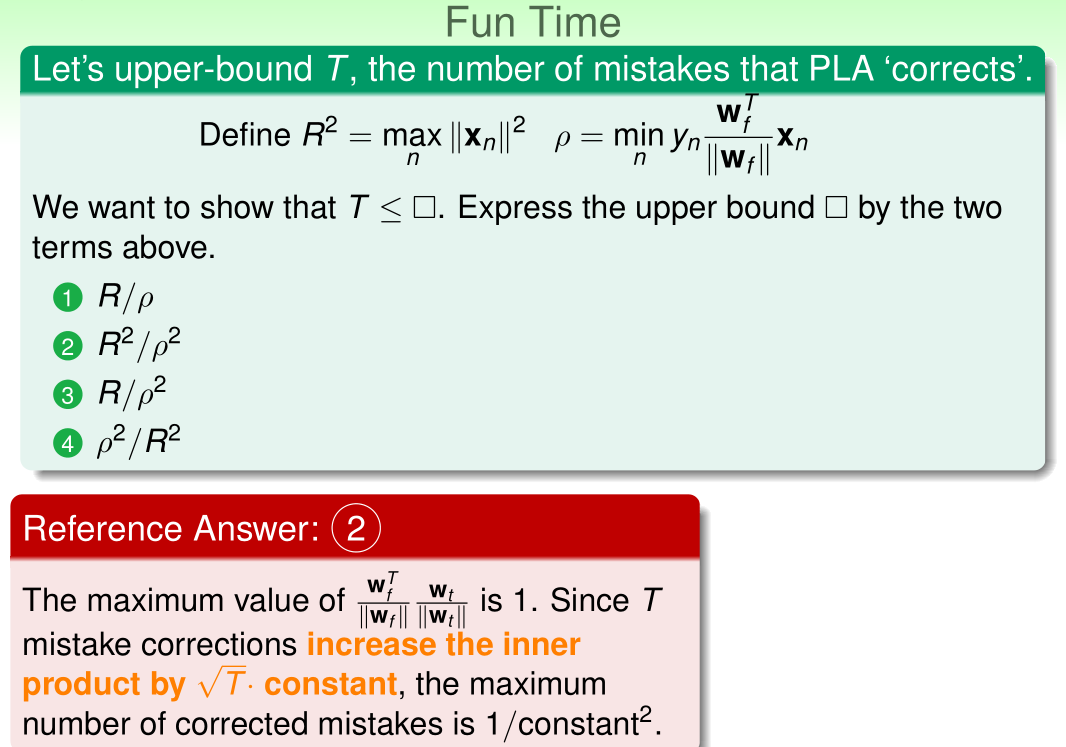#### 2.4 Non-Separable Data

• Guarantee: as long as linear separable and correct by mistake
• inner product of $\mathbf{w}_f$ and $\mathbf{w}_t$ grows fast; length of $\mathbf{w}_t$ grows slowly
• PLA “lines” are more and more aligned with $\mathbf{w}_f$ $\Rightarrow$ halts
• Pros: simple to implement, fast, works in any dimension $d$
• Cons:
• “assumes” linear separable $\cal D$ to halt
• property unknown in advance (no need for PLA if we know $\mathbf{w}_f$)
• not fully sure how long halting takes ($\rho$ depends on $\mathbf{w}_f$)
• though practically fast
• What if $\cal D$ not linear separable?
• Learning with Noisy Data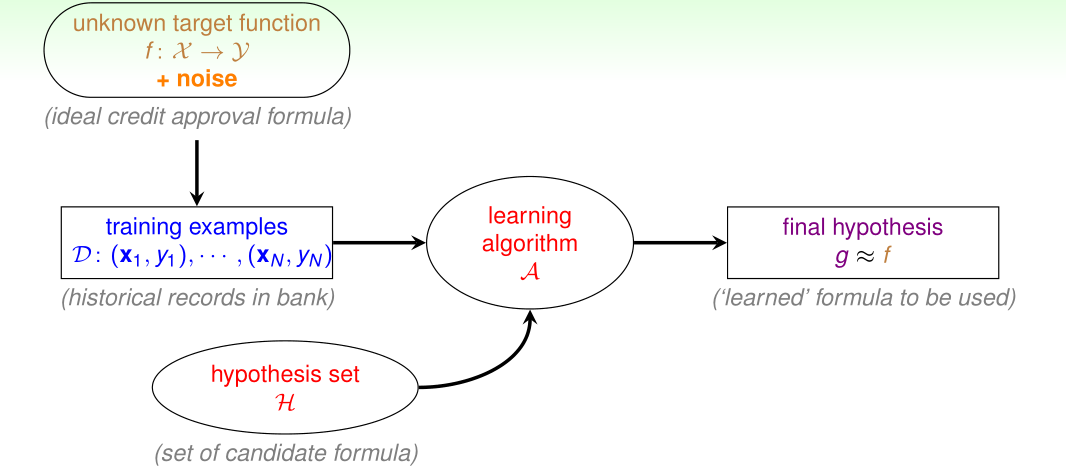• how to at least get $g \approx f$ on noisy $\cal D$?
• Line with noise tolerance

• assume little noise: $y_n = f(\mathbf{x}_n)$ usually

• if so, $g \approx f$ on $\cal D$ $\Leftrightarrow$ $y_n=g(\mathbf{x}_n)$ usually

• How about $\mathbf{w}_g \leftarrow \mathop{\text{argmin}}_{\mathbf{w}} \sum_{n=1}^N \left[ y_{n} \neq \operatorname{sign}\left(\mathbf{w}^{T} \mathbf{x}_n\right) \right]$

• NP-hard to solve, unfortunately
• can we modify PLA to get an approximately good $g$?

• Pocket Algorithm

• Modify PLA algorithm (black lines) by keeping best weights in pocket

• Initialize pocket weights $\mathbf{\hat{w}}$

• find a (random) mistake of $\mathbf{w}t$, called $\left(\mathbf{x}{n(t)}, y_{n(t)}\right)$

• (try to) correct the mistake by $\mathbf{w}_{t+1} \leftarrow \mathbf{w}_t + y_{n(t)}\mathbf{x}_{n(t)}$

• if $\mathbf{w}{t+1}$ makes fewer mistakes that $\mathbf{\hat{w}}$, replace $\mathbf{\hat{w}}$ by $\mathbf{w}{t+1}$

• … until enough iterations

• return $\mathbf{\hat{w}}$ (called $\mathbf{w}_{\text{POCKET}}$) as $g$

QUIZ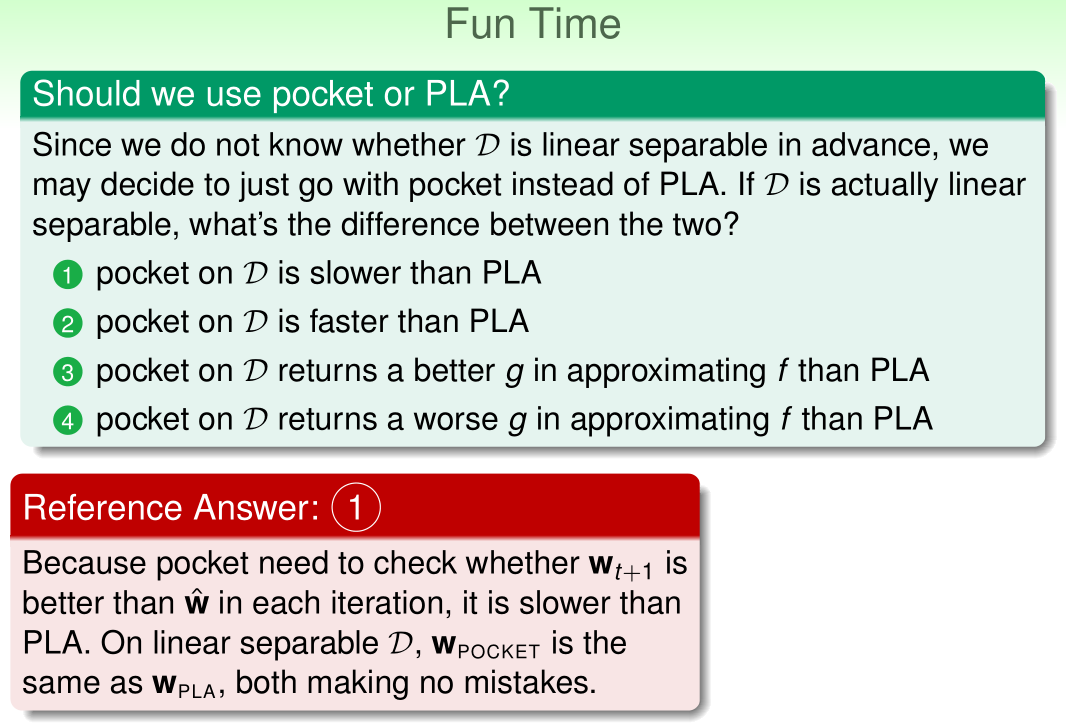## 3 Types of Learning

Learning comes with many possibilities in different applications, with our focus being binary classification or regression from a batch of supervised data with concrete features …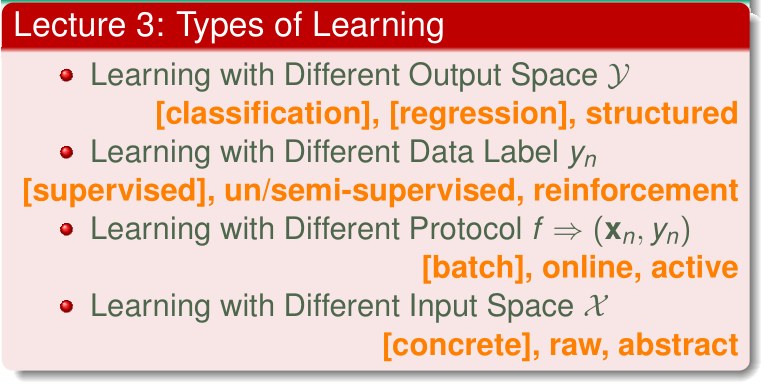#### 3.1 Learning with Different Output Space

• More binary classification problems

• like:
• credit approve/disapprove
• email spam/non-spam
• patient sick/not sick
• core and important problem with many tools as building block of other tools
• Multiclass classification

• coin recognition problem

• classify US coins (1c, 5c, 10c, 25c) by (size, mass)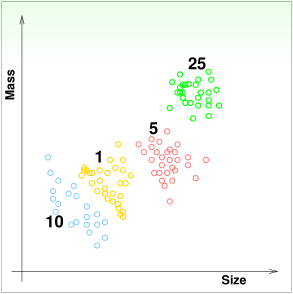• $\mathcal{Y} = \lbrace 1, 5, 10, 25\rbrace$
• binary classification: special case with $K = 2$

• Other multiclass classification problems:

• written digits $\Rightarrow$ 0, 1, 2, …, 9
• pictures $\Rightarrow$ apple, orange, strawberry, …
• emails $\Rightarrow$ spam, primary, social, promotion, update …
• many applications in practice, especially for recognition

• Regression:

• patient recovery prediction problem
• binary classification: patient features $\Rightarrow$ sick or not
• multiclass classification: patient features $\Rightarrow$ which type of cancer
• regression: patient features $\Rightarrow$ how many days before recovery
• $\mathcal{Y}=\Bbb{R}$ or $\mathcal{Y}=[\text{lower},\text{upper}]$ $\subset \Bbb{R}$ (bounded regression)
• deeply studied in statistics
• Other regression problems:
• company data $\Rightarrow$ stock price
• climate data $\Rightarrow$ temperature
• also core and important with math statistical tools as building block of other tools
• Structured Learning: sequence tagging problem

• e.g. NLP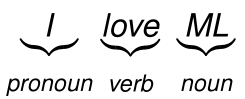• multiclass classification $\Rightarrow$ word class
• structured learning:
• sentence $\Rightarrow$ structure (class of each word)
• $\mathcal{Y} = \lbrace PVN, PVP, NVN, PV, \cdots \rbrace$, not including $VVVVVV$
• huge multiclass classification problem (structure $\equiv$ hyperclass) without explicit class definition
• Other structured learning problems:

• protein data $\Rightarrow$ protein folding
• speech data $\Rightarrow$ speech parse tree
• a fancy but complicated learning problem

• Mini Summary: learning with different output space $\cal Y$

• binary classification: $\mathcal{Y} = \lbrace -1, +1 \rbrace$
• multiclass classification: $\mathcal{Y} = \lbrace 1, 2, \cdots, K \rbrace$
• regression: $\mathcal{Y} = \Bbb{R}$
• structured learning: $\mathcal{Y} = \text{structures}$
• … and a lot more!
QUIZ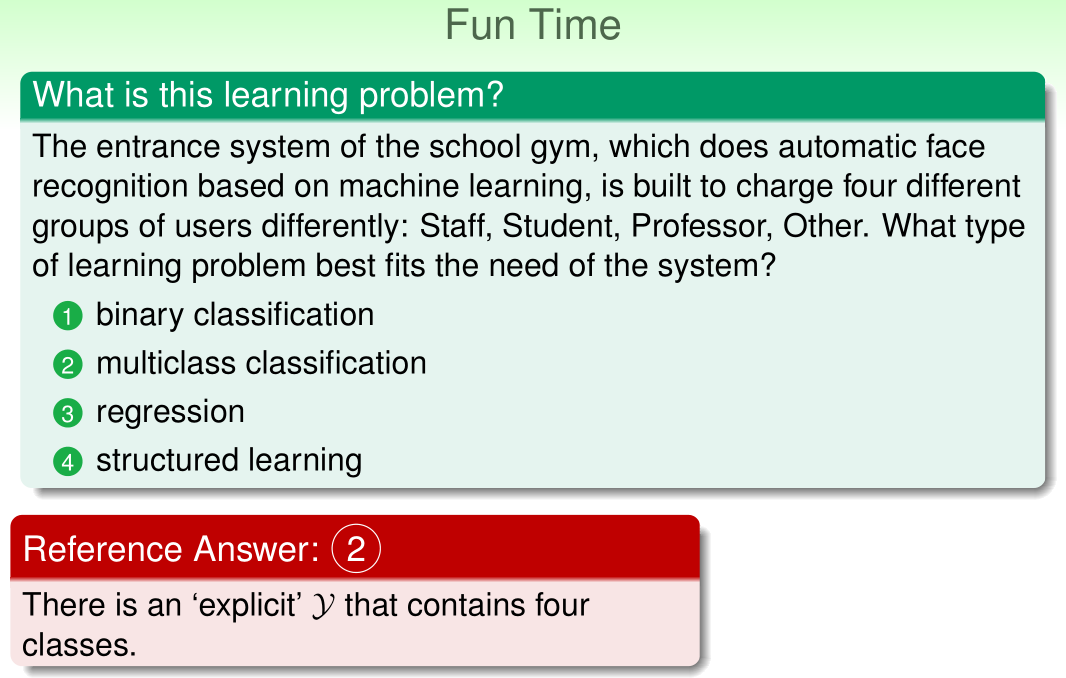#### 3.2 Learning with Different Data Label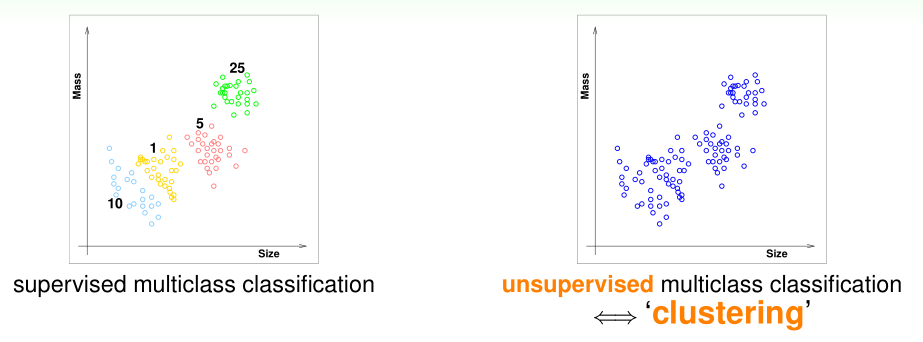• Supervised Learning

• coin recognition revisited
• supervised multiclass classification
• Unsupervised Learning

• coin recognition without $y_n$
• unsupervised multiclass classification $\Leftrightarrow$ clustering
• Other clustering problems:
• articles $\Rightarrow$ topics
• consumer profiles $\Rightarrow$ consumer groups
• clustering: a challenging but useful problem
• Other unsupervised learning problems

• clustering: $\lbrace \mathbf{x}_n \rbrace \Rightarrow \text{cluster}(\mathbf{x})$
• $\approx$ unsupervised multiclass classification
• density estimation: $\lbrace \mathbf{x}_n \rbrace \Rightarrow \text{density}(\mathbf{x})$
• $\approx$ unsupervised bounded regression
• e.g., traffic reports with location $\Rightarrow$ dangerous areas
• outlier detection: $\lbrace \mathbf{x}_n \rbrace \Rightarrow \text{unusual}(\mathbf{x})$
• $\approx$ extreme unsupervised binary classification
• e.g., Internet logs $\Rightarrow$ intrusion alert
• … and a lot more!
• Semi-supervised Learning: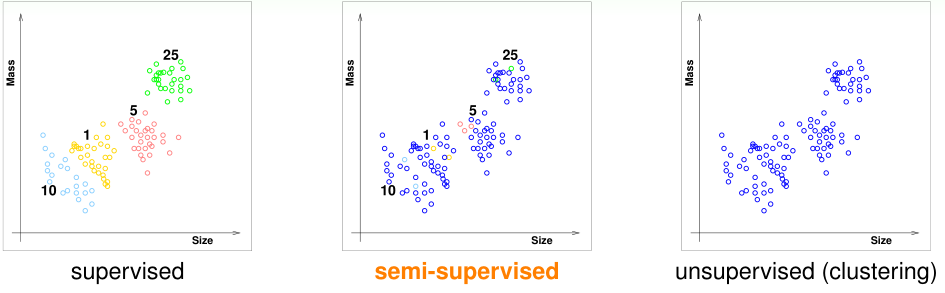• coin regression with some $y_n$
• Other semi-supervised learning problems
• face images with a few labeled $\Rightarrow$ face identifier
• medicine data with a few labeled $\Rightarrow$ medicine effect predictor
• leverage unlabeled data to avoid expensive labeling
• Reinforcement Learning: a very different but natural way of learning

• Teach your dog: say “sit down”
• if
• The dog pees on the ground
• BAD DOG. THAT’S A VERY WRONG ACTION.
• The dog sits down.
• Good Dog. Let me give you some cookies
• cannot easily show the dog that $y_n$ = “sit”, when $\mathbf{x}_n$ = “sit down”
• but can “punish” to say $\tilde{y}_n$ = “pee is wrong”
• but can “reward” to say $\tilde{y}_n$ = “sit is good”
• Other RL problems using $(\mathbf{x}, \tilde{y}, \text{goodness})$
• (customer, ad choice, ad click earning) $\Rightarrow$ ad system
• (cards, strategy, winning amount) $\Rightarrow$ black jack agent
• RL: learn with partial/implicit information (often sequentially)
• Mini Summary: learning with different data label $y_n$

• supervised: all $y_n$
• unsupervised: no $y_n$
• semi-supervised: some $y_n$
• reinforcement: implicit $y_n$ by goodness ($\tilde{y}_n$)
• … and more!
QUIZ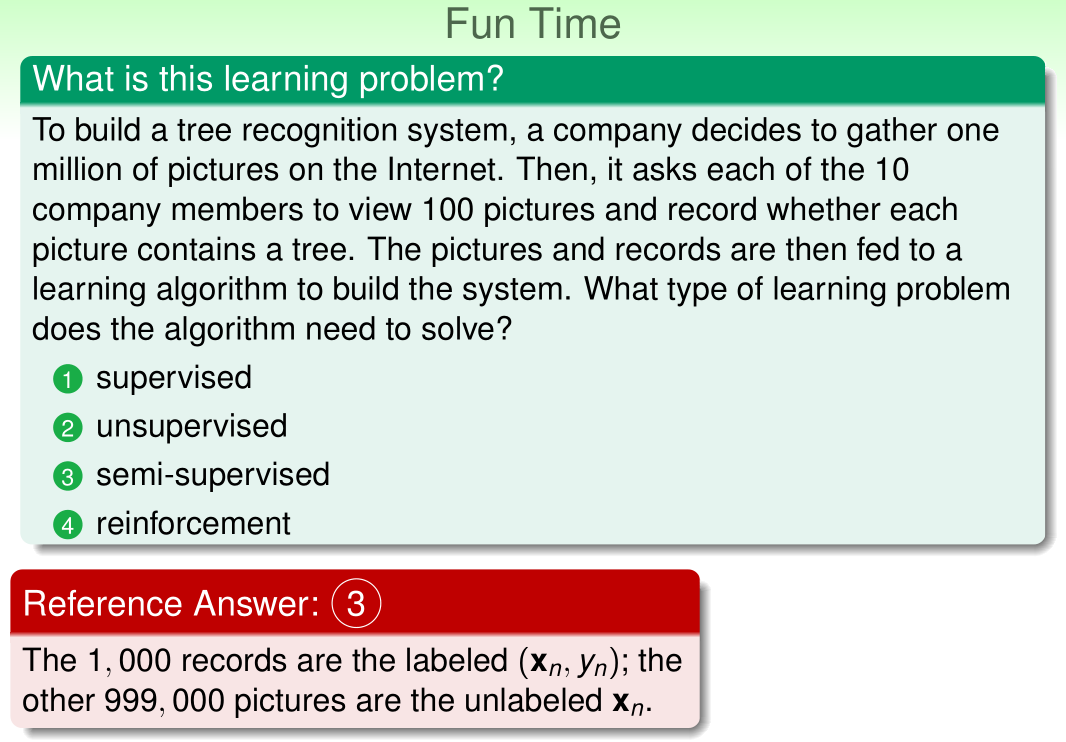#### 3.3 Learning with Different Protocol

• Batch Learning:
• coin recognition revisited
• batch supervised multiclass classification: learn from all known data
• More batch learning problems
• batch of (email,spam?) $\Rightarrow$ spam filter
• batch of (patient,cancer) $\Rightarrow$ cancer classifier
• batch of patient data $\Rightarrow$ group of patients
• Online Learning:
• spam filter that improve
• batch spam filter:
• learn with known (email, spam?) pairs, and predict with fixed $g$
• online spam filter, which sequentially:
• observe an email $\mathbf{x}_t$
• predict spam status with current $g_t(\mathbf{x}_t)$
• receive desired label $y_t$ from user, and then update $g_t$ with $(\mathbf{x}_t, y_t)$
• Connection to what we’ve learned
• PLA can be easily adapted to online protocol (how?)
• RL learning is often done online (why?)
• Online learning: hypothesis improves through receiving data instances sequentially
• Active Learning: learning by asking
• protocol $\Leftrightarrow$ learning philosophy
• batch: “duck feeding”
• online: “passive sequential”
• query the $y_n$ of the chosen $\mathbf{x}_n$
• active: improve hypothesis with fewer labels (hopefully) by asking questions strategically
• Mini Summary: learning with different protocol $\Rightarrow$ $(\mathbf{x}_n, y_n)$
• batch: all known data
• online: sequential (passive) data
• active: strategically-observed data
• … and more!
QUIZ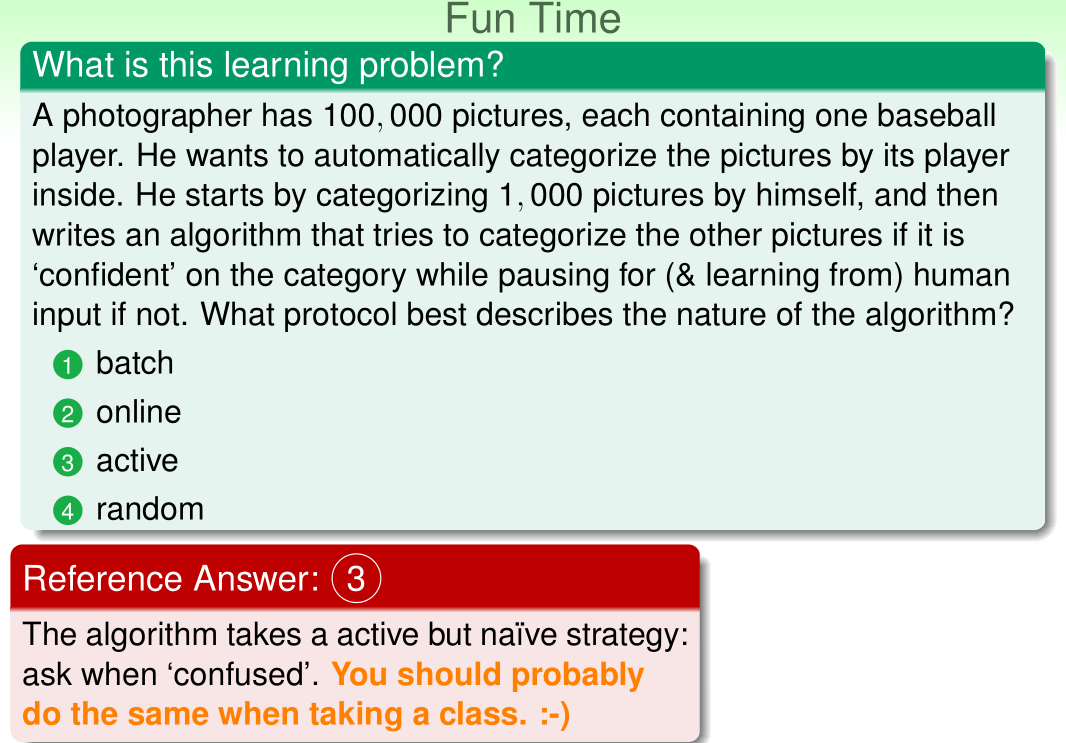#### 3.4 Learning with Different Input Space

• Concrete features: each dimension of $\cal{X} \subseteq \Bbb{R}^d$

• More on concrete features
• (size, mass) for coin classification
• customer info for credit approval
• patient info for cancer diagnosis
• often including human intelligence on the learning task
• concrete features: the easy ones for ML
• Raw features:

• digit recognition problem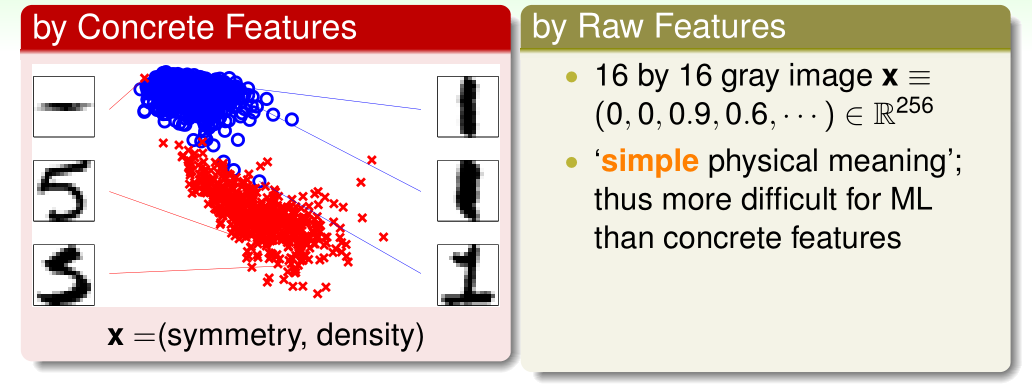• features $\Rightarrow$ meaning of digit
• a typical supervised multiclass classification problem
• Other problems with raw features

• image pixels, speech signal, etc.
• raw features: often need human or machines to convert to concrete ones

• Abstract features

• rating prediction problem
• given previous (userid, itemid, rating) tuples, predict the rating that some userid would give to itemid?
• a regression problem with $\cal{Y} \subseteq \Bbb R$ as rating and $\mathcal{X} \subseteq N \times N$ as (userid, itemid)
• no physical meaning; thus even more difficult for ML
• Other problems with abstract features
• student ID in online tutoring system
• abstract: again need feature conversion/extraction/construction
• Mini Summary: Learning with different space $\cal X$

• concrete: sophisticated (and related) physical meaning
• raw: simple physical meaning
• abstract: no (or little) physical meaning
• … and more!
QUIZ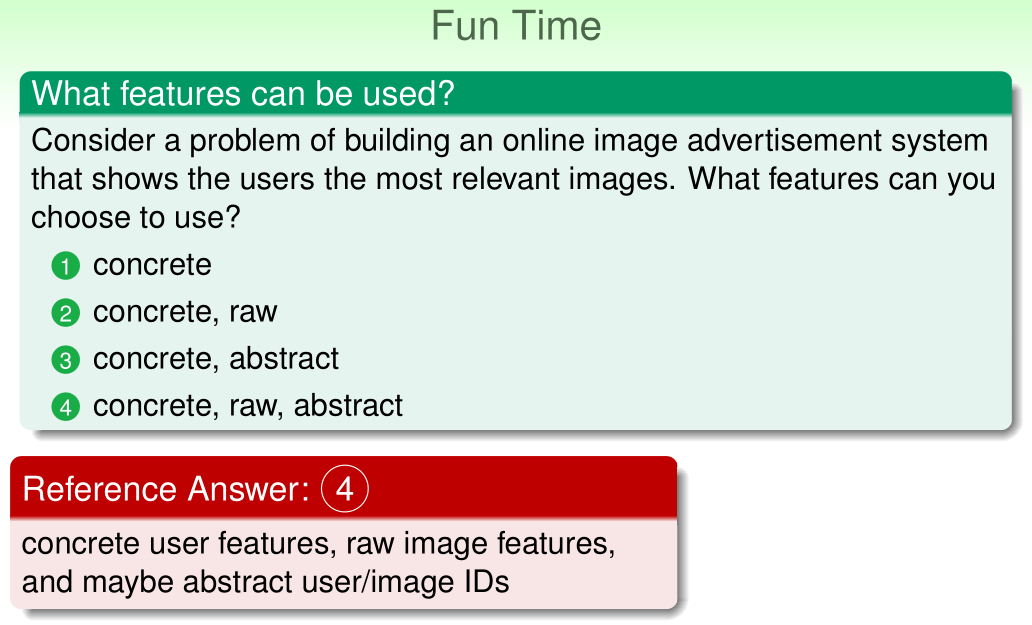## 4 Feasibility of Learning

Learning can be “probably approximately correct” when given enough statistical data and finite number of hypotheses …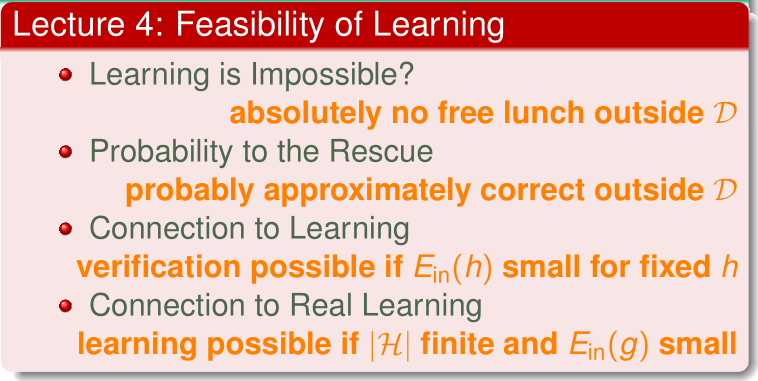#### 4.1 Learning is Impossible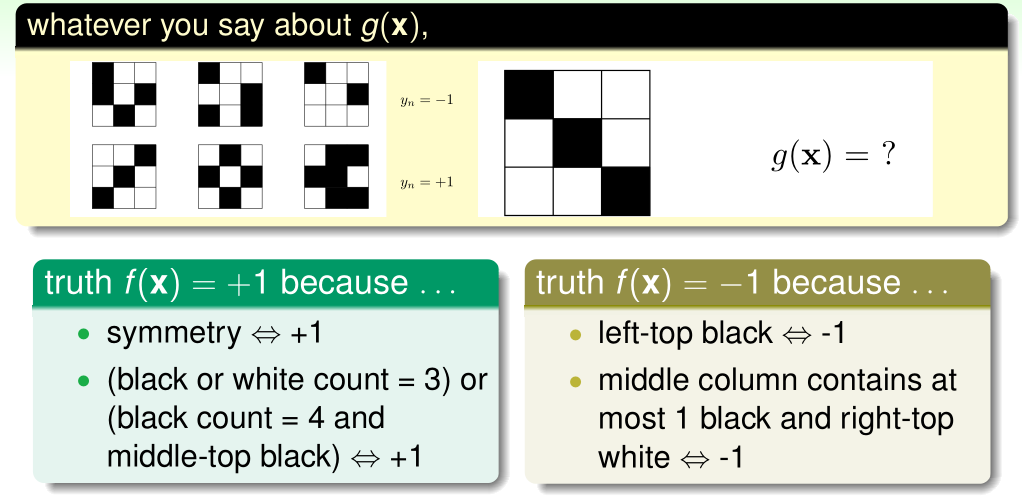• all valid reasons, your adversarial teacher can always call you didn’t learn. :cry:
• A simple binary classification problem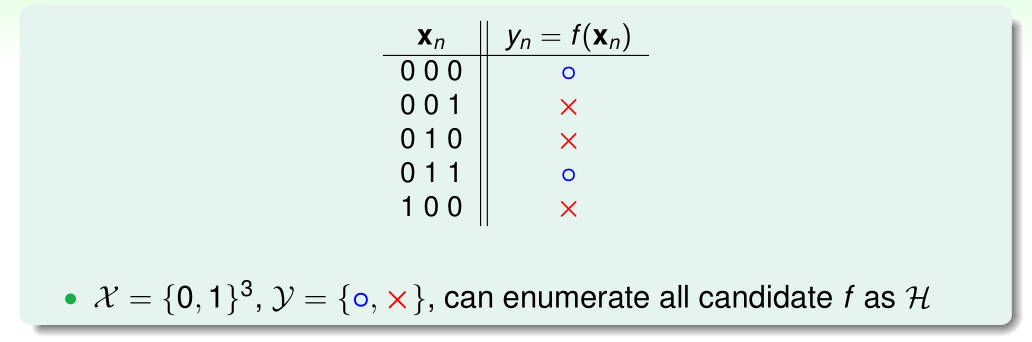• pick $g \in \cal{H}$ with all $g(\mathbf{x}_n)=y_n$ (like PLA), does $g \approx f$?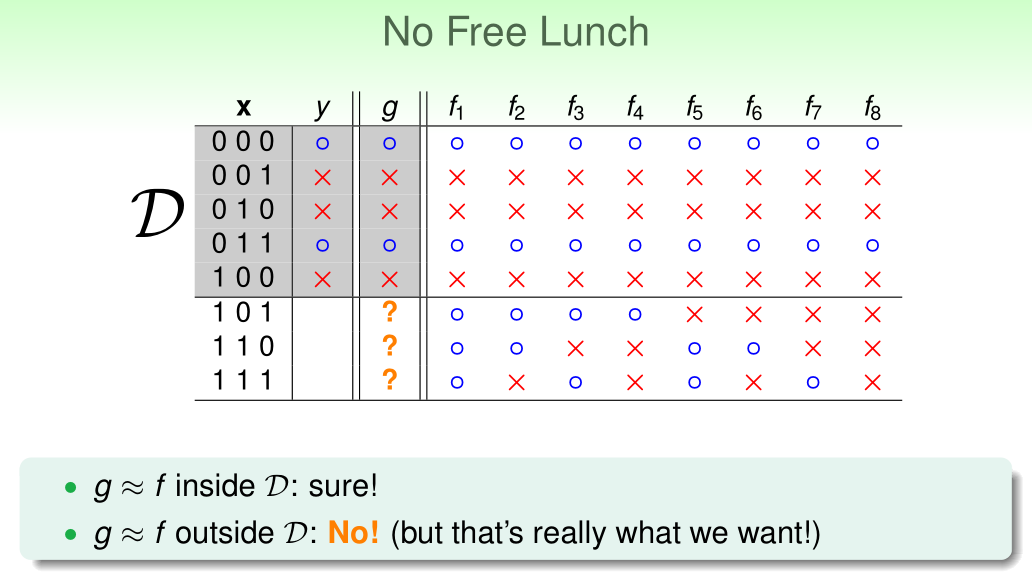• learning from $\cal D$ (to infer something outside $\cal D$) is doomed if any unknown $f$ can happen. :cry:
QUIZ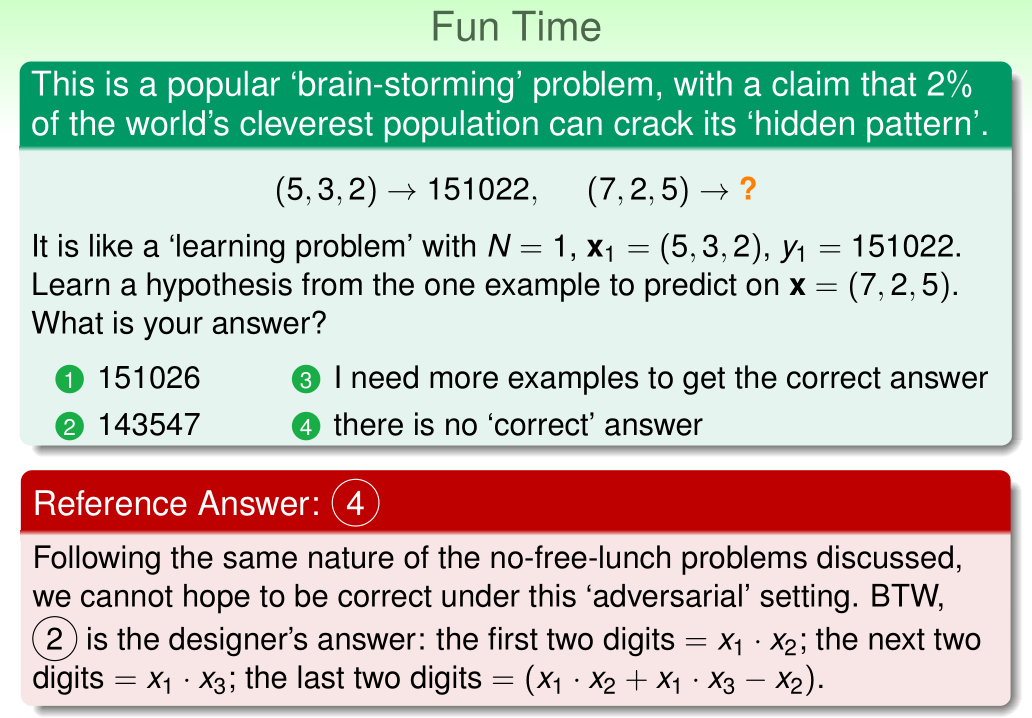#### 4.2 Probability to the Rescue

• Inferring something unknown

• difficult to infer unknown target $f$ outside $\cal D$ in learning; can we infer something unknown in other scenarios?
• consider a bin of many many orange and green marbles
• do we know the orange portion (probability)> No!
• can you infer the orange probability?
• Statistics 101: Inferring orange probability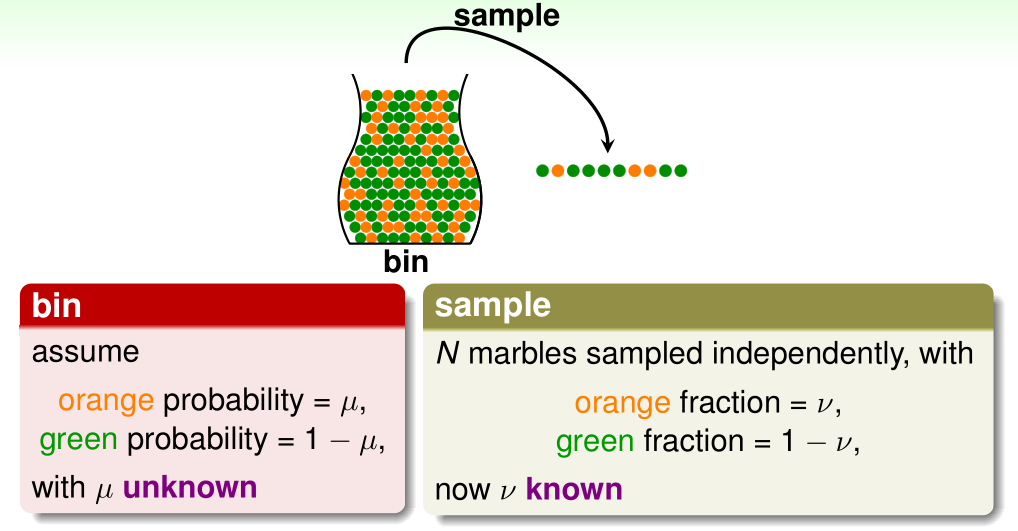• Possible versus Probable: does in-sample $v$ say anything about out-of-sample $\mu$?
• possibly not: sample can be mostly green while bin is mostly orange
• probably yes: in-sample $\nu$ likely close to unknown $\mu$
• formally, what does $\nu$ say about $\mu$?
• Hoeffding’s Inequality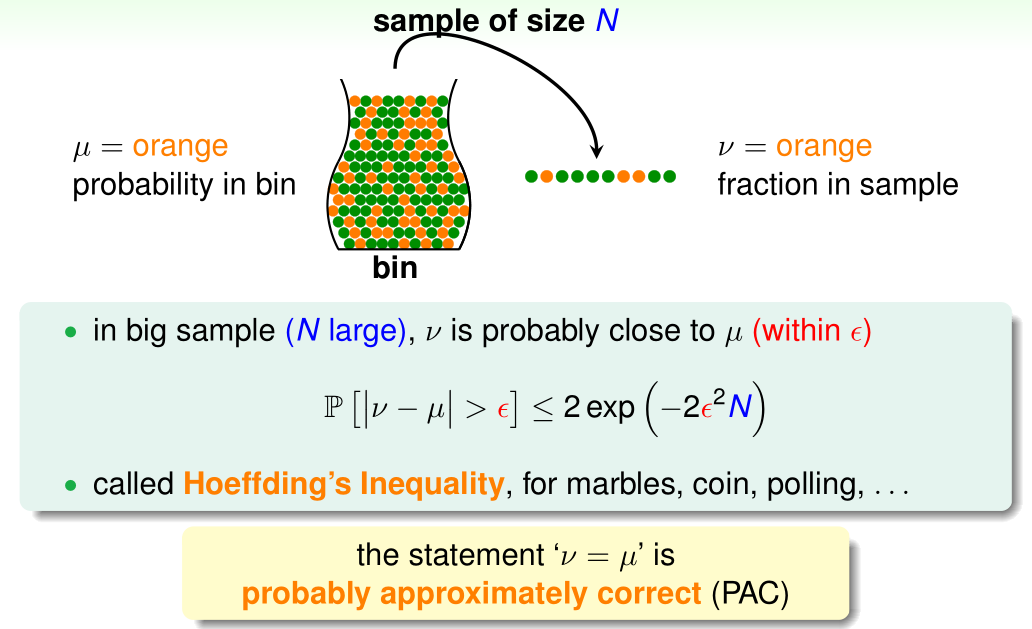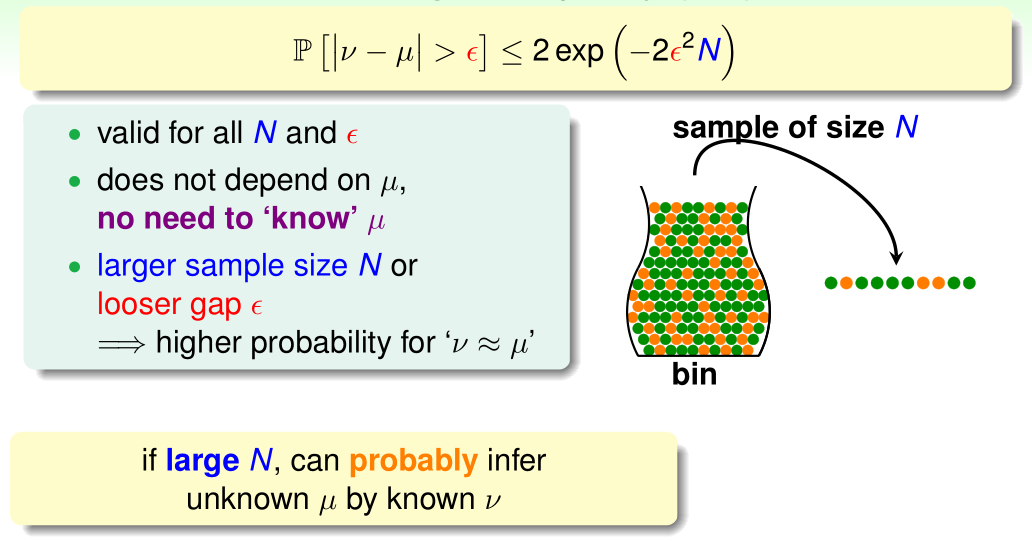• $\epsilon$: tolerance
QUIZ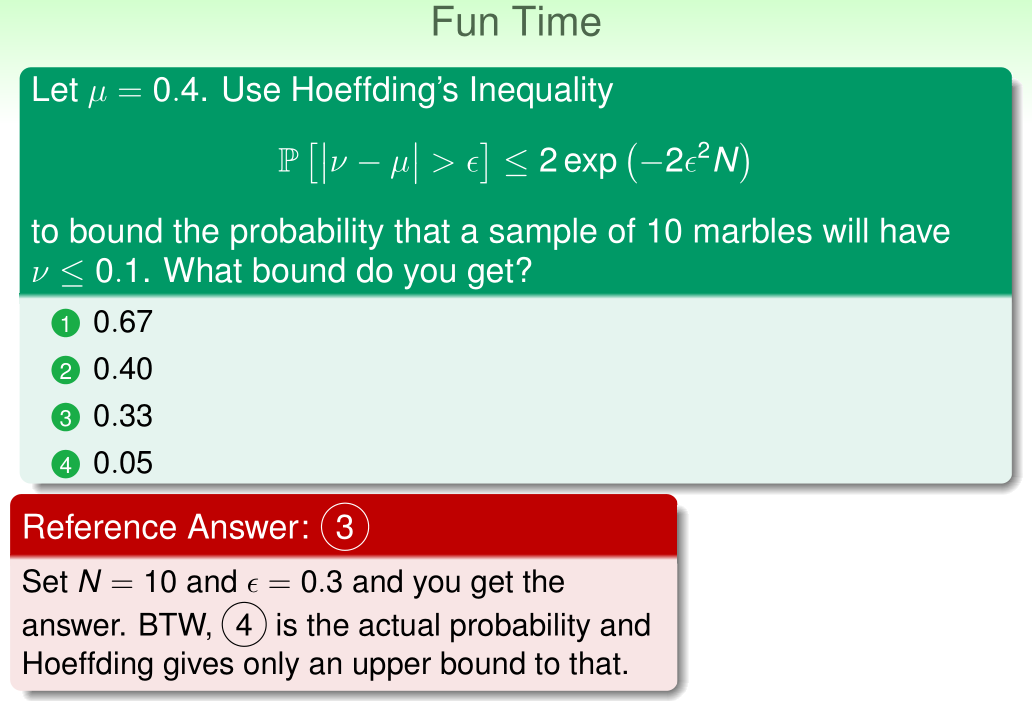#### 4.3 Connection to Learning

• Connection to Learning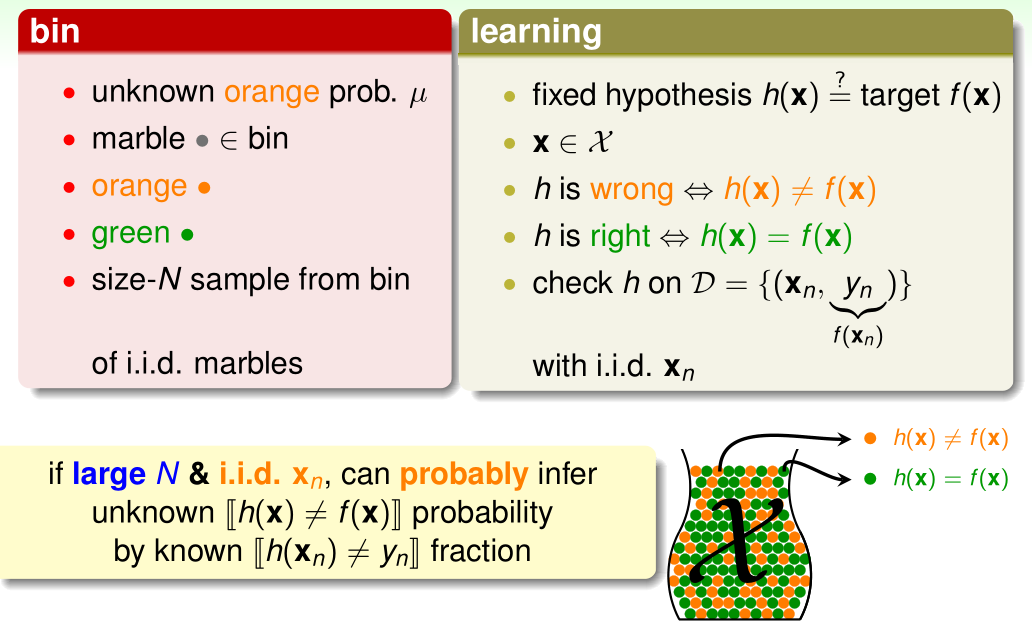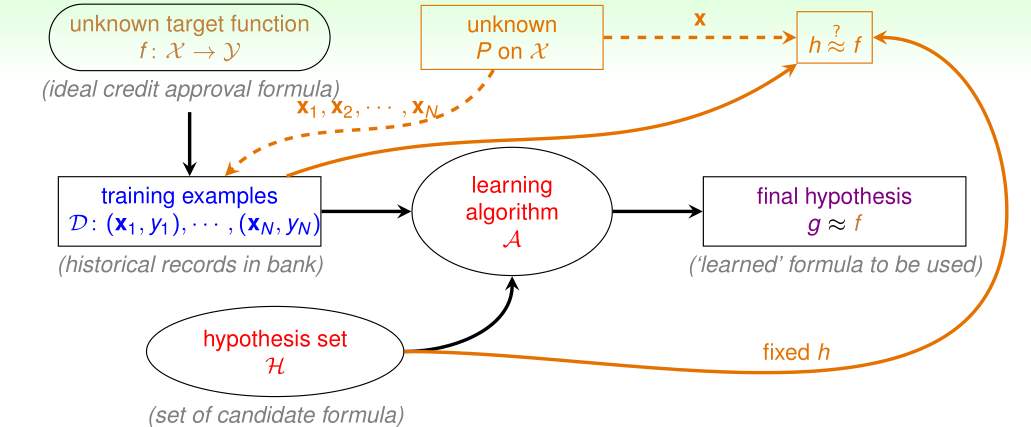• for any fixed $h$, can probably infer
• unknown $E_{out}(h) = \mathop{\varepsilon}_\limits{\mathbf{x}\sim P} [h(\mathbf{x}) \neq f(\mathbf{x})]$ (out-of-sample error)
• by known $E_{in}(h) = \frac{1}{N} \sum_{n=1}^N [h(\mathbf{x}_n) \neq y_n]$ (in-sample error)
• The formal guarantee

• for any fixed $h$, in big data ($N$ large), in-sample error $E_{in}(h)$ is probably close to out-of-sample error $E_{out}(h)$ (within $\epsilon$) $\Bbb{R} \left[|E_{in}(h) - E_{out}(h)| > \epsilon \right] \leq 2 \exp(-2\epsilon^2N)$

• same as the “bin” analogy

• valid for all $N$ and $\epsilon$
• does not depend on $E_{out}(h)$, no need to “know” $E_{out}(h)$
• $f$ and $P$ can stay unknown
• if $E_{in}(h) \approx E_{out}(h)$ and $E_{in}(h)$ small $\Rightarrow$ $E_{out}(h)$ small $\Rightarrow$ $h \approx f$ with respect to $P$

• Verification of one $h$

• for any fixed $h$, when data large enough, $E_{in}(h) \approx E_{out}(h)$
• can we claim “good learning” ($g \approx f)$?
• Yes!
• if $E_{in}(h)$ is small for the fixed $h$ and $\cal A$ pick the $h$ as $g$
• $\Rightarrow$ $g = f$ PAC
• No!
• if $\cal A$ forced to pick THE $h$ as $g$
• $\Rightarrow$ $E_{in}(h)$ almost always not small
• $\Rightarrow$ $g \ne f$ PAC!
• real learning:
• $\cal A$ shall make choices $\in \cal H$ (like PLA)
• rather than being forced to pick one $h$
• The verification flow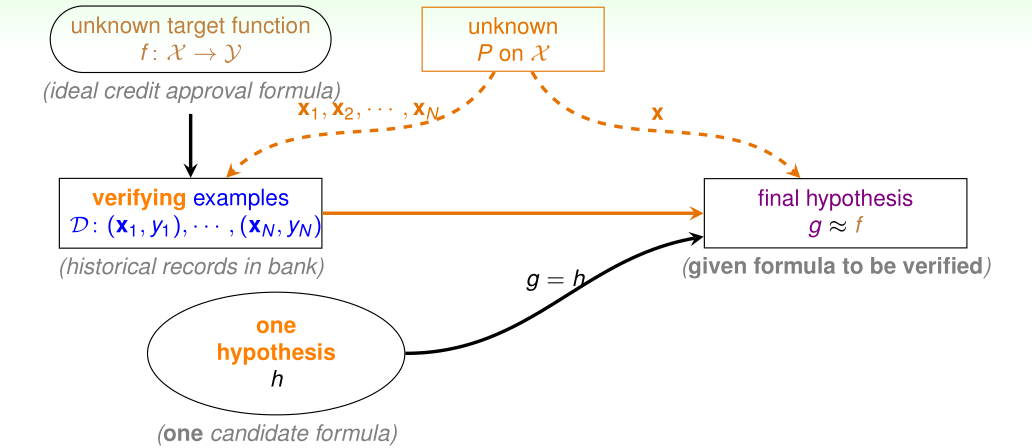• can now use historical records (data) to verify “one candidate formula” $h$
QUIZ#### 4.4 Connection to Real Learning

• Multiple $h$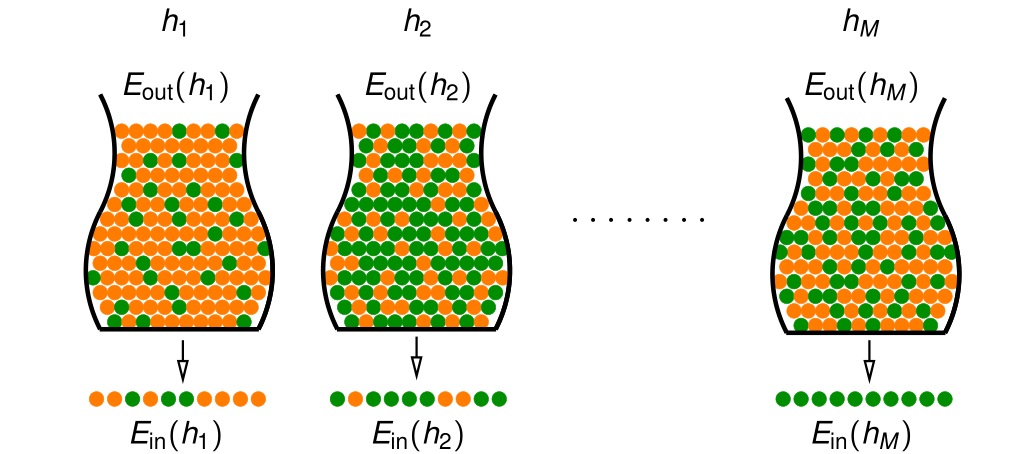• real learning (say like PLA):
• BINGO when getting all green marbles?
• Coin game

• Q: if everyone in size-150 NTU ML class flips a coin 5 times, and one of the students gets 5 heads for her coin $g$. Is $g$ really magical?
• A: No. Even if all coins are fair, the probability that one of the coins results in 5 heads is $1 - \left(\frac{31}{32}\right)^{150} > 99\%$.
• BAD sample: $E_{in}$ and $E_{out}$ far away
• can get worse when involving choice

• e.g., $E_{out} = \frac{1}{2}$, but getting all heads ($E_{in} = 0$)!
• BAD Data for One $h$

• $E_{out}(h)$ and $E_{in}(h)$ far away
• e.g., $E_{out}(h)$ big (far from $f$), but $E_{in}$ small (correct on most examples)
• Hoeffding: $\Bbb{P}_{\cal D}[{\color{Orange}{\text{BAD }}} \mathcal{D} \text{ for } h] \le \ …$

• BAD Data for Many $h$

• $\Leftrightarrow$ no “freedom of choice” by $\cal A$
• $\Leftrightarrow$ there exists some $h$ such that $E_{out}(h)$ and $E_{in}(h)$ far away
• for $M$ hypothesis, bound of $\Bbb{P}_{\cal D}[{\color{Orange}{\text{BAD }}} \mathcal{D} ]$?
• Bound of BAD Data %

• finite-bin version of Hoeffding, valid for all $M$, $N$ and $\epsilon$
• does not depend on any $E_{out} (h_m )$, no need to “know” $E_{out }(h_m)$
• $f$ and $P$ can stay unknown
• $E_{in}(g) = E_{out}(g)$ is PAC, regardless of $\cal A$
• most reasonable $\cal A$ (like PLA/pocket):
• pick the $h_m$ with lowest $E_{in}(h_m )$ as $g$
• The Statistical Learning Flow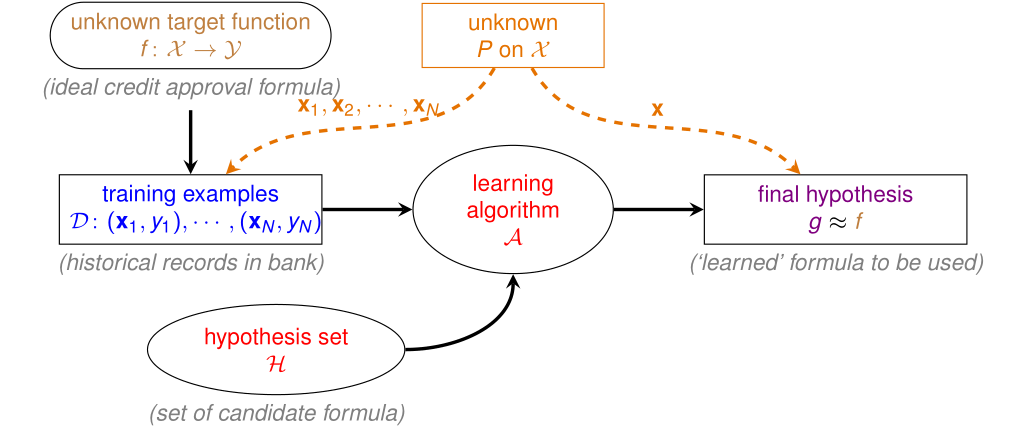• if $\vert \mathcal{H} \vert= M$ finite, $N$ large enough
• for whatever $g$ picked by $\cal A$, $E_{out}(h) \approx E_{in}(g)$
• if $\cal A$ finds one $g$ with $E_{in}(g) \approx 0$,
• PAC guarantee for $E_{out}(g) \approx 0$ $\Rightarrow$ learning possible :happy:
• if $M = \infty$ (like perceptrons)?
• see you in the next lectures
QUIZ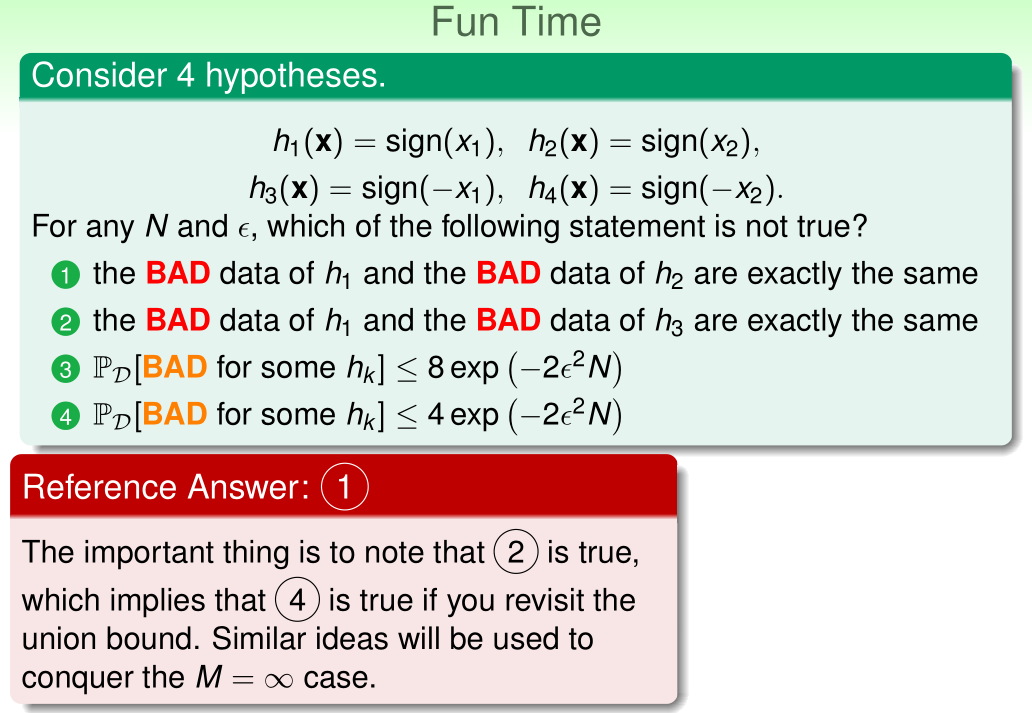Thistledown